Courses

# Instrumentation Engineering - IN 2012 GATE Paper (Practice Test)

## 65 Questions MCQ Test GATE Past Year Papers for Practice (All Branches) | Instrumentation Engineering - IN 2012 GATE Paper (Practice Test)

Description
This mock test of Instrumentation Engineering - IN 2012 GATE Paper (Practice Test) for GATE helps you for every GATE entrance exam. This contains 65 Multiple Choice Questions for GATE Instrumentation Engineering - IN 2012 GATE Paper (Practice Test) (mcq) to study with solutions a complete question bank. The solved questions answers in this Instrumentation Engineering - IN 2012 GATE Paper (Practice Test) quiz give you a good mix of easy questions and tough questions. GATE students definitely take this Instrumentation Engineering - IN 2012 GATE Paper (Practice Test) exercise for a better result in the exam. You can find other Instrumentation Engineering - IN 2012 GATE Paper (Practice Test) extra questions, long questions & short questions for GATE on EduRev as well by searching above.
QUESTION: 1

Solution:
QUESTION: 2

Solution:
QUESTION: 3

### One of the parts (A, B, C, D) in the sentence given below contains an ERROR. Which one of the following is INCORRECT? I requested that he should be given the driving test today instead of tomorrow.

Solution:
QUESTION: 4

Which one of the following options is the closest in meaning to the word given below?

Latitude

Solution:
QUESTION: 5

Choose the most appropriate word from the options given below to complete the following sentence:

Given the seriousness of the situation that he had to face, his ___ was impressive.

Solution:
QUESTION: 6

Q. 6 - Q. 10 carry two marks each.

Q. Raju has 14 currency notes in his pocket consisting of only Rs. 20 notes and Rs. 10 notes. The total
money value of the notes is Rs. 230. The number of Rs. 10 notes that Raju has is

Solution:
QUESTION: 7

One of the legacies of the Roman legions was discipline. In the legions, military law prevailed and discipline was brutal. Discipline on the battlefield kept units obedient, intact and fighting, even when the odds and conditions were against them.

Which one of the following statements best sums up the meaning of the above passage?

Solution:
QUESTION: 8

A and B are friends. They decide to meet between 1 PM and 2 PM on a given day. There is a condition that whoever arrives first will not wait for the other for more than 15 minutes. The probability that they will meet on that day is

Solution:
QUESTION: 9

The data given in the following table summarizes the monthly budget of an average household.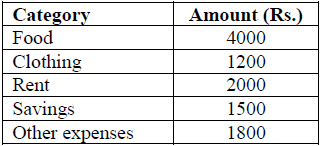The approximate percentage of the monthly budget NOT spent on savings is

Solution:
QUESTION: 10

There are eight bags of rice looking alike, seven of which have equal weight and one is slightly heavier. The weighing balance is of unlimited capacity. Using this balance, the minimum number of weighings required to identify the heavier bag is

Solution:
QUESTION: 11

Q. 1 – Q. 25 carry one mark each.

Q. If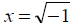, then the value of xx is

Solution:
QUESTION: 12

With initial condition x(1) = 0.5 , the solution of the differential equation,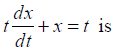Solution:
QUESTION: 13

Two independent random variables X and Y are uniformly distributed in the interval [–1, 1]. The probability that max[X, Y] is less than 1/2 is

Solution:
QUESTION: 14

The unilateral Laplace transform of f (t) is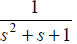. The unilateral Laplace transform of  tf(t) is

Solution:
QUESTION: 15

Given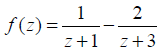. If C is a counterclockwise path in the z-plane such that |z +1| =1, the value of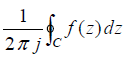is

Solution:
QUESTION: 16

The average power delivered to an impedance (4 - j3)? by a current 5cos(100πt +100) A is

Solution:
QUESTION: 17

In the circuit shown below, the current through the inductor is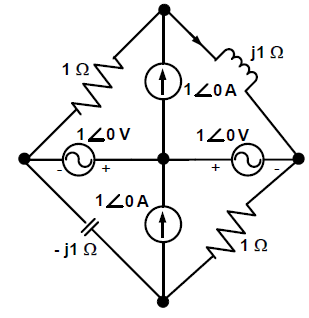Solution:
QUESTION: 18

I n the following figure, C1 and C2 are ideal capacitors. C1 has been charged to 12 V before the ideal
switch S is closed at t = 0. The current i(t) for all t is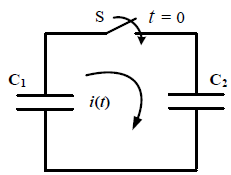Solution:
QUESTION: 19

T he impedance looking into nodes 1 and 2 in the given circuit is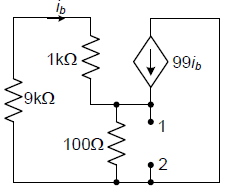Solution:
QUESTION: 20

T he i-v characteristics of the diode in the circuit given below are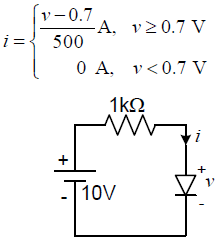The current in the circuit is

Solution:
QUESTION: 21

A system with transfer function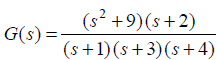is excited by sin(ω t) . The steady-state output of the system is zero at

Solution:
QUESTION: 22

The output Y of a 2-bit comparator is logic 1 whenever the 2-bit input A is greater than the 2-bit input B. The number of combinations for which the output is logic 1, is

Solution:
QUESTION: 23

In the sum of products function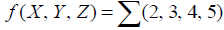, the prime implicants are

Solution:
QUESTION: 24

Consider the given circuit.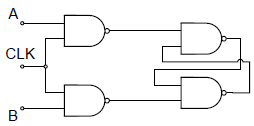In this circuit, the race around

Solution:
QUESTION: 25

If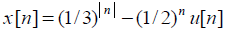, then the region of convergence (ROC) of its Z-transform in the Z-plane will be

Solution:
QUESTION: 26

A capacitive motion transducer circuit is shown. The gap d between the parallel plates of the capacitor is varied as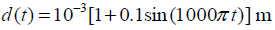. If the value of the capacitance is 2pF at
t = 0 ms, the output voltage Vo at t = 2 ms is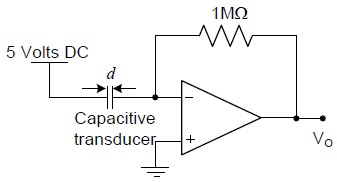Solution:
QUESTION: 27

A psychrometric chart is used to determine

Solution:
QUESTION: 28

A strain gauge is attached on a cantilever beam as shown. If the base of the cantilever vibrates according to the equation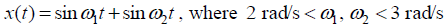, then the output of the strain gauge is proportional to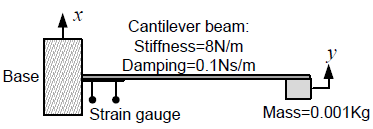Solution:
QUESTION: 29

T he transfer function of a Zero-Order-Hold system with sampling interval T is

Solution:
QUESTION: 30

A n LED emitting at 1 μm with a spectral width of 50 nm is used in a Michelson interferometer. To
obtain a sustained interference, the maximum optical path difference between the two arms of the
interferometer is

Solution:
QUESTION: 31

L ight of wavelength 630 nm in vacuum, falling normally on a biological specimen of thickness 10 μm, splits into two beams that are polarized at right angles. The refractive index of the tissue for the two polarizations are 1.32 and 1.333. When the two beams emerge, they are out of phase by

Solution:
QUESTION: 32

The responsivity of the PIN photodiode shown is 0.9 A/W. To obtain Vout of –1 V for an incident optical power of 1 mW, the value of R to be used is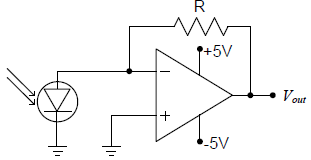Solution:
QUESTION: 33

A periodic voltage waveform observed on an oscilloscope across a load is shown. A permanent magnet moving coil (PMMC) meter connected across the same load reads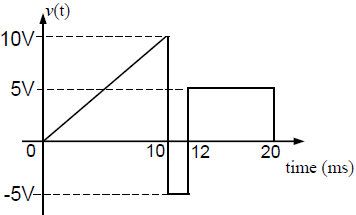Solution:
QUESTION: 34

For the circuit shown in the figure, the voltage and current expressions are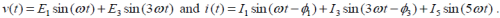The average power measured by the Wattmeter is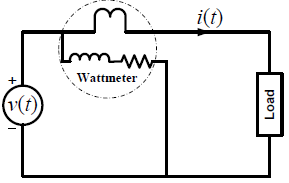Solution:
QUESTION: 35

The bridge method commonly used for finding mutual inductance is

Solution:
QUESTION: 36

Q. 36 - Q 65 carry two marks each:

A fair coin is tossed till a head appears for the first time. The probability that the number of required tosses is odd, is

Solution:
QUESTION: 37

Given that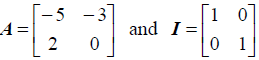the value of A3 is

Solution:
QUESTION: 38

The direction of vector A is radially outward from the origin, with |A| = krn where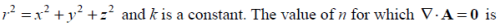Solution:
QUESTION: 39

The maximum value of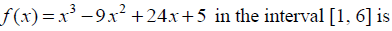Solution:
QUESTION: 40

Consider the differential equation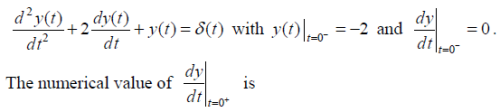Solution:
QUESTION: 41

If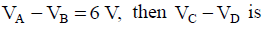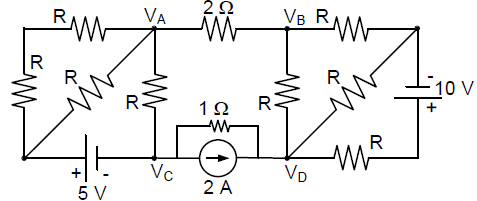Solution:
QUESTION: 42

A ssuming both the voltage sources are in phase, the value of R for which maximum power is transferred from circuit A to circuit B is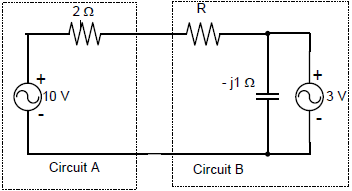Solution:
QUESTION: 43

The voltage gain Av of the circuit shown below is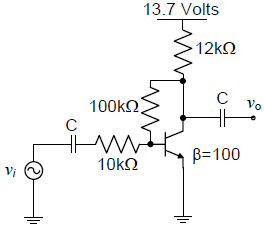Solution:
QUESTION: 44

The state variable description of an LTI system is given by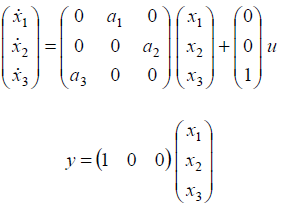where y is the output and u is the input. The system is controllable for

Solution:
QUESTION: 45

The state transition diagram for the logic circuit shown is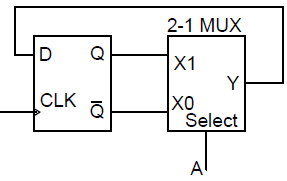Solution:
QUESTION: 46

The Fourier transform of a signal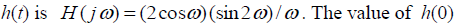is

Solution:
QUESTION: 47

L et y[n] denote the convolution of h[n] and g[n], where h[n] = (1/2)n u[n] and g[n] is a causal sequence. If y = 1 and y = 1/2, then g equals

Solution:
QUESTION: 48

The feedback system shown below oscillates at 2 rad/s when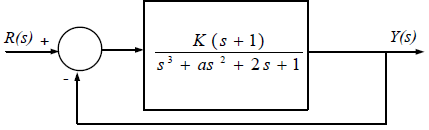Solution:
QUESTION: 49

The circuit shown is a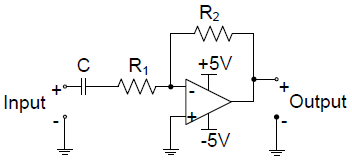Solution:
QUESTION: 50

The input x(t) and output y(t) of a system are related as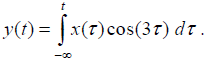The system is

Solution:
QUESTION: 51

A double convex lens is used to couple a laser beam of diameter 5 mm into an optical fiber with a numerical aperture of 0.5. The minimum focal length of the lens that should be used in order to focus the entire beam into the fiber is

Solution:
QUESTION: 52

A n analog voltmeter uses external multiplier settings. With a multiplier setting of 20 kilo-ohms it reads
440 V and with a multiplier setting of 80 kilo-ohms it reads 352 V. For a multiplier setting of 40 kilo-ohms the

Solution:
QUESTION: 53

The open loop transfer function of a unity negative feedback control system is given by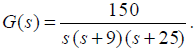.The gain margin of the system is

Solution:
QUESTION: 54

A dynamometer arm makes contact with the piezoelectric load cell as shown. The g-constant of the piezoelectric material is 50 x 10-3Vm/N and the surface area of the load cell is 4 cm2. If a torque τ =20 Nm is applied to the dynamometer, the output voltage Vo of the load cell is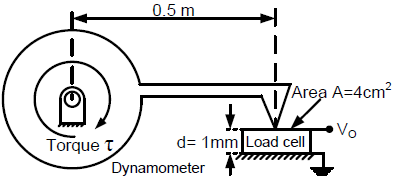Solution:
QUESTION: 55

Water (density: 1000 kgm–3) stored in a cylindrical drum of diameter 1 m is emptied through a horizontal pipe of diameter 0.05 m. A pitot-static tube is placed inside the pipe facing the flow. At the time when the difference between the stagnation and static pressures measured by the pitotstatic tube is 10 kPa, the rate of reduction in water level in the drum is,

Solution:
QUESTION: 56

A U-tube manometer of tube diameter D is filled with a liquid of zero viscosity. If the volume of the liquid filled is V, the natural frequency of oscillations in the liquid level about its mean position, due to small perturbations, is

Solution:
QUESTION: 57

The open loop transfer function of a unity gain negative feedback control system is given by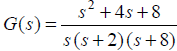. The angle θ , at which the root locus approaches the zeros of the system,
satisfies

Solution:
QUESTION: 58

Common Data Questions

Common Data for Questions 58 and 59:

With 10 V dc connected at port A in the linear nonreciprocal two-port network shown below, the following
were observed:

(i) 1 ohm connected at port B draws a current of 3 A

(ii) 2.5 ohm connected at port B draws a current of 2 A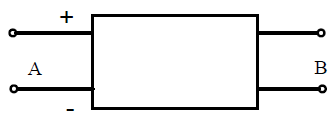Q. With 10 V dc connected at port A, the current drawn by 7 ohm connected at port B is

Solution:
QUESTION: 59

Common Data Questions

Common Data for Questions 58 and 59:

With 10 V dc connected at port A in the linear nonreciprocal two-port network shown below, the following
were observed:

(i) 1 ohm connected at port B draws a current of 3 A

(ii) 2.5 ohm connected at port B draws a current of 2 AQ. For the same network, with 6 V dc connected at port A, 1 ohm connected at port B draws 7/3 A.
If 8 V dc is connected to port A, the open circuit voltage at port B is

Solution:
QUESTION: 60

Common Data for Questions 60 and 61:

The deflection profile y (x) of a cantilever beam due to application of a point force F (in Newton), as a
function of distance x from its base, is given by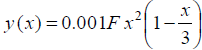. The angular deformation θ at the end of the cantilever is measured by reflecting a laser beam off a mirror M as shown in the figure.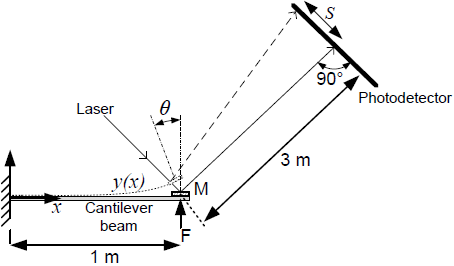Q. The translation S of the spot of laser light on the photodetector when a force of F =1 N is applied
to the cantilever is

Solution:
QUESTION: 61

Common Data for Questions 60 and 61:

The deflection profile y (x) of a cantilever beam due to application of a point force F (in Newton), as a
function of distance x from its base, is given by. The angular deformation θ at the end of the cantilever is measured by reflecting a laser beam off a mirror M as shown in the figure.If linear variable differential transformers (LVDTs) are mounted at x = 1/2 m and x = 1/4 m on the
cantilever to measure the effect of time varying forces, the ratio of their outputs is

Solution:
QUESTION: 62

The transfer function of a compensator is given as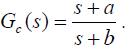Q. Gc(s) is a lead compensator if

Solution:
QUESTION: 63

The transfer function of a compensator is given asQ. The phase of the above lead compensator is maximum at

Solution:
QUESTION: 64

In the circuit shown, the three voltmeter readings are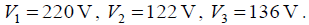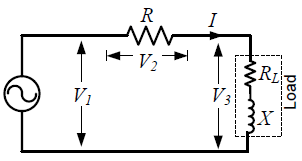Q. The power factor of the load is

Solution:
QUESTION: 65

In the circuit shown, the three voltmeter readings are